# OECD Tourism flows data analysis 2 - Data wrangling and one variable visualization with R.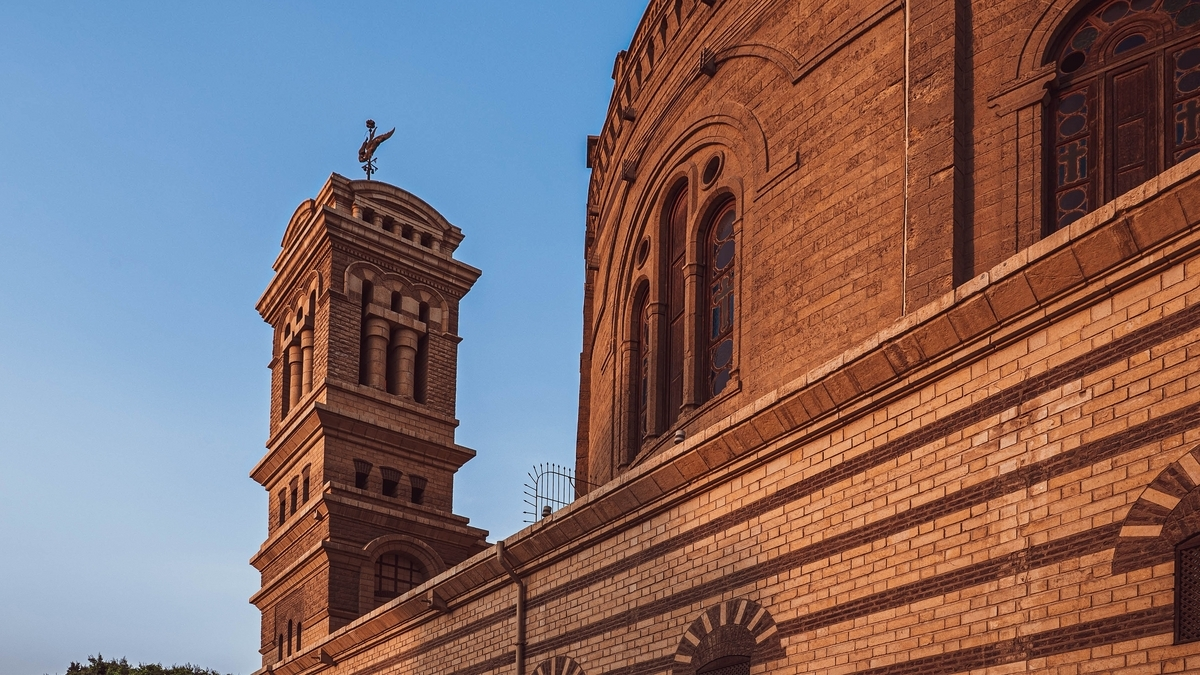Unsplash2H Mediaが撮影した写真

This post is following of the above post.

I make ACC_NIGHTS only data frame with filter() function in R.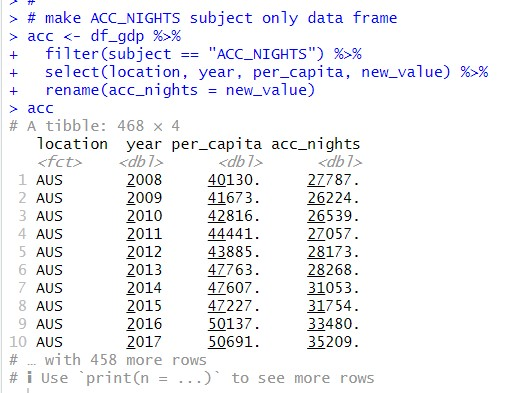Next, I make INTER_ARR only data frame.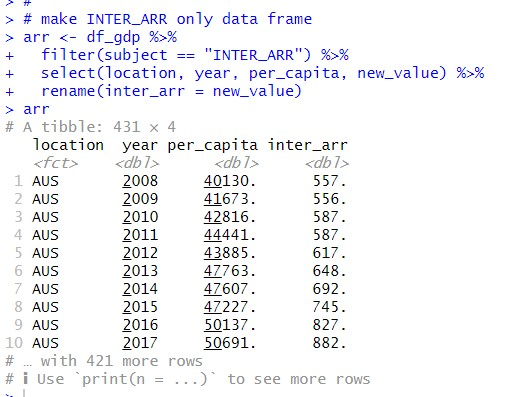I make INTER_DEP only data frame.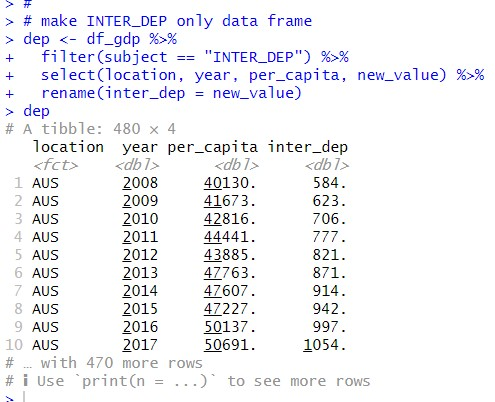Then, I merge those three data frames into one data frame using inner_join() function.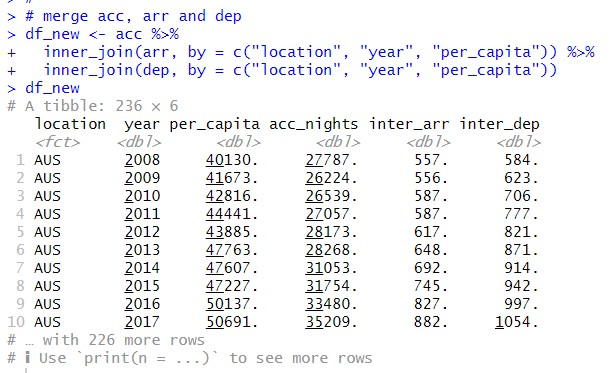I will analyze df_new data frame.
Let's see summary statistics with summary() function.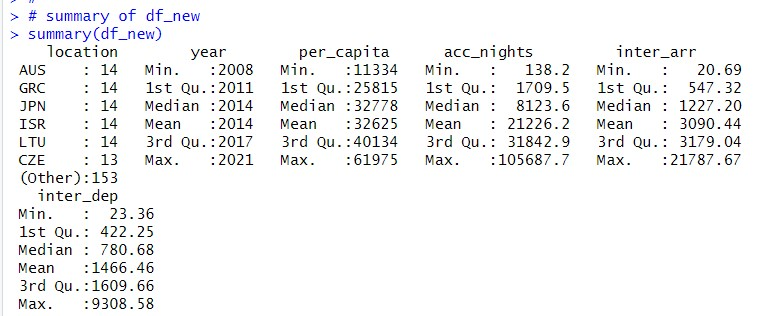I see AUS, GRC, JPN, ISR and LTU have 14 observations. Year starts from 2008 to 2021.

per_capita varies from 11224 to 61975, mean is 32625.

acc_nights varies from 138.2 to 10568.7, mean is 21226.2.

inter_arr varies from 20.69 to 21787.67, mean is 2090.44.

inter_dep varies from 23.36 to 9308.58, mean is 1466.46.

Let's visualize each variables.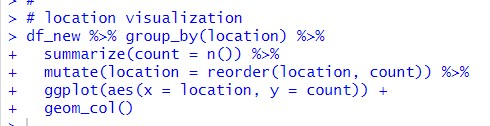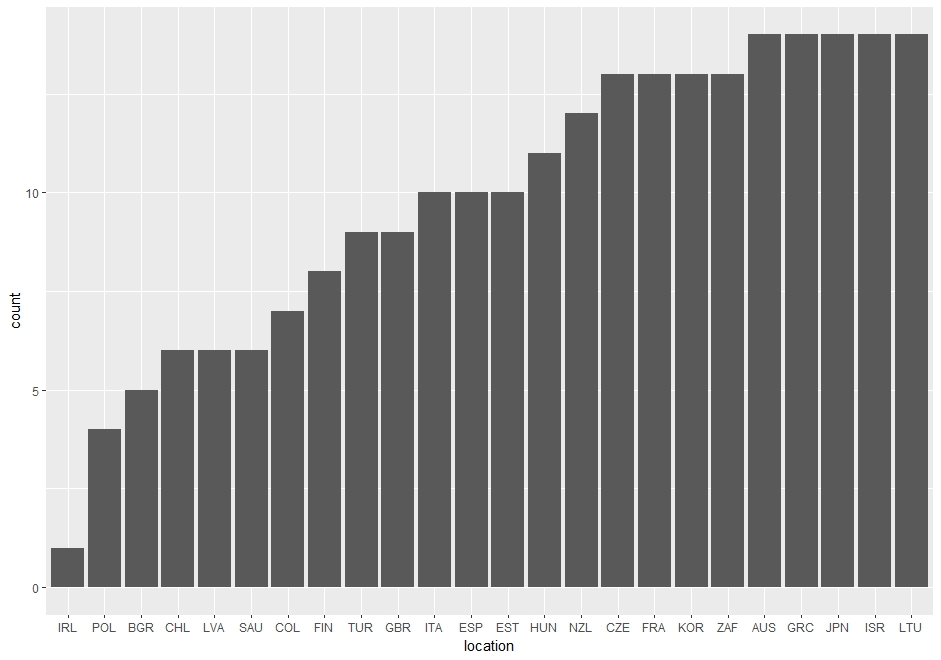Since location is a categorical variable, I use group_by() and summarize() function to count number of observations of locations, then I use geom_col().

Next, year.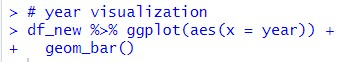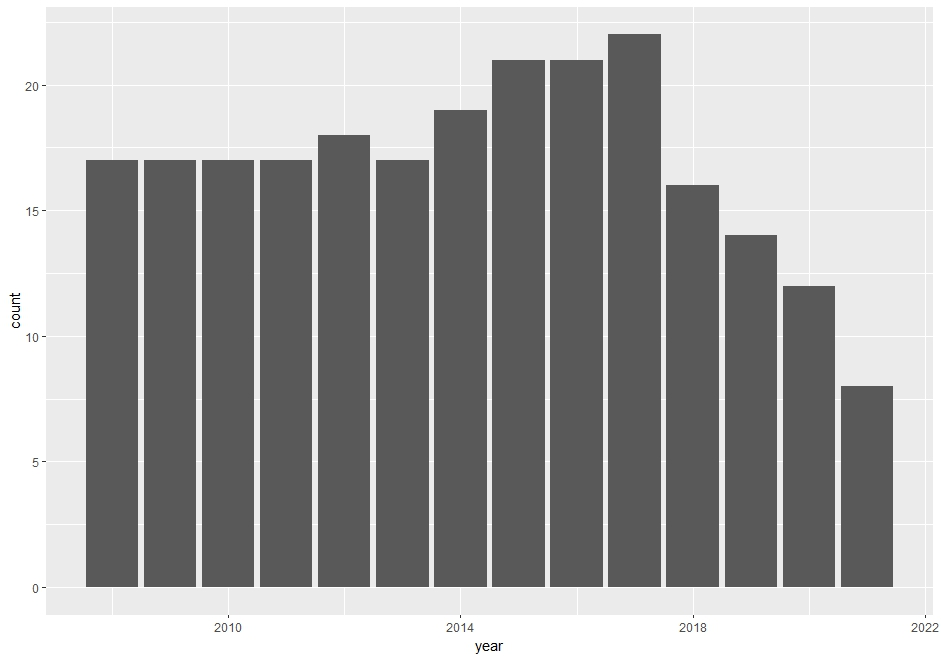I use geom_bar() function to count number of observations of year, I see 2017 has the most observations.

Let's go on with per_capita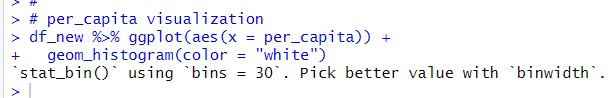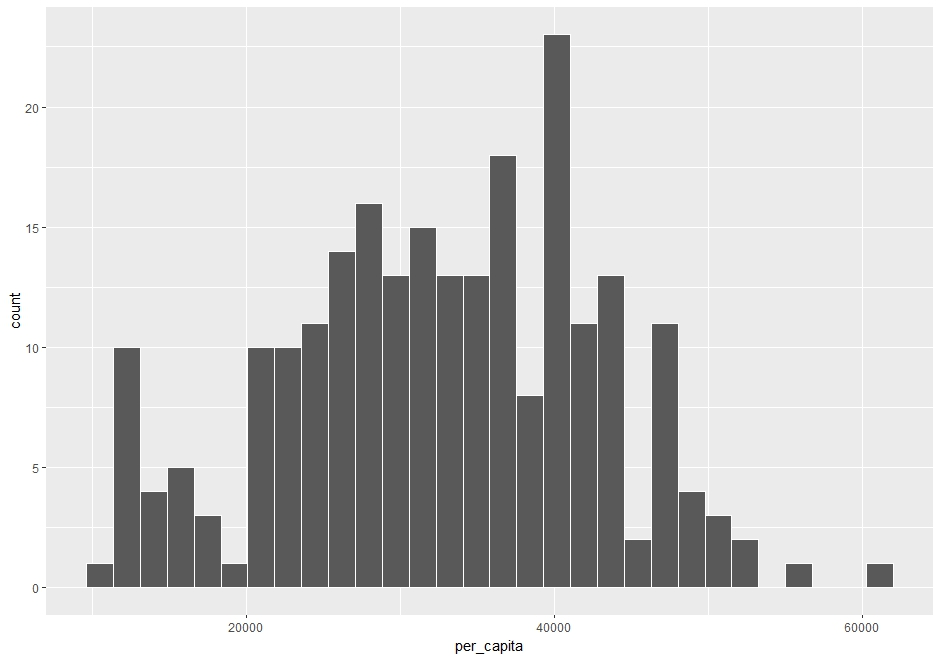Since per_capita is a numerical variable, I use geom_histogram() function for drawing a histogram.

Next, acc_nights.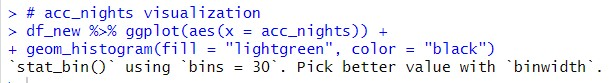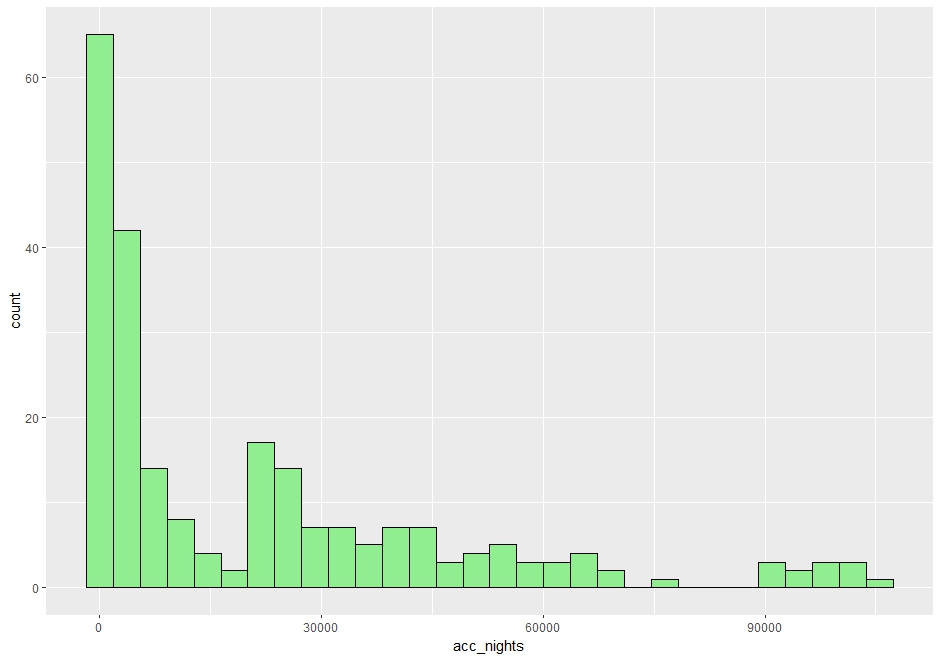I see acc_nights are not normaly distributed. it is very skewed to the right direction.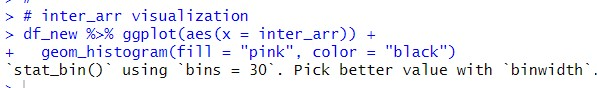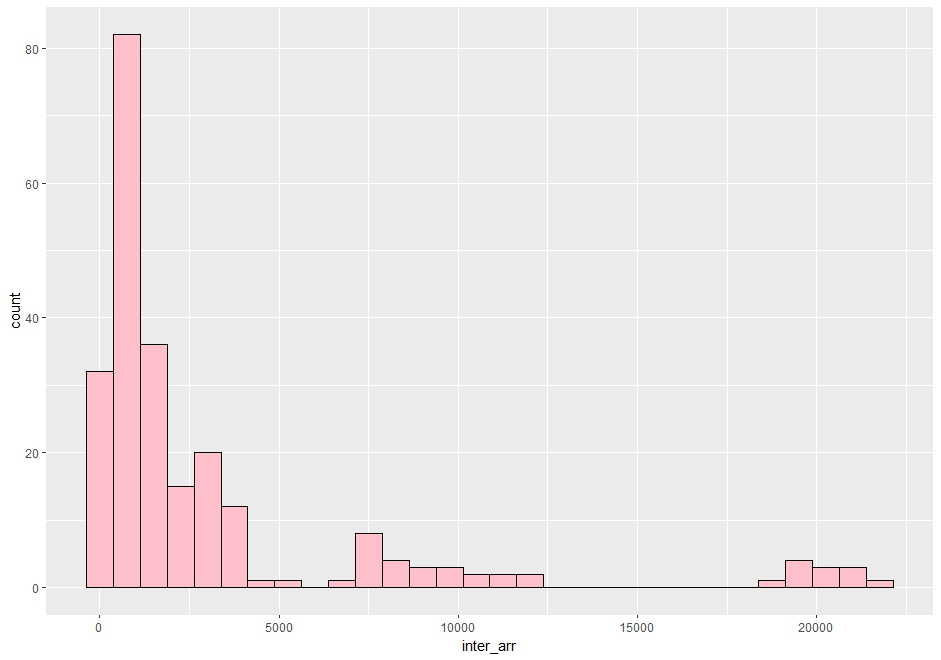inter_arr are also skewd.

Last, let's check inter_dep.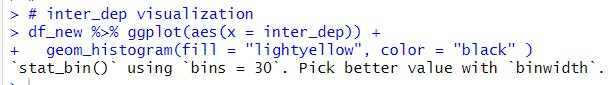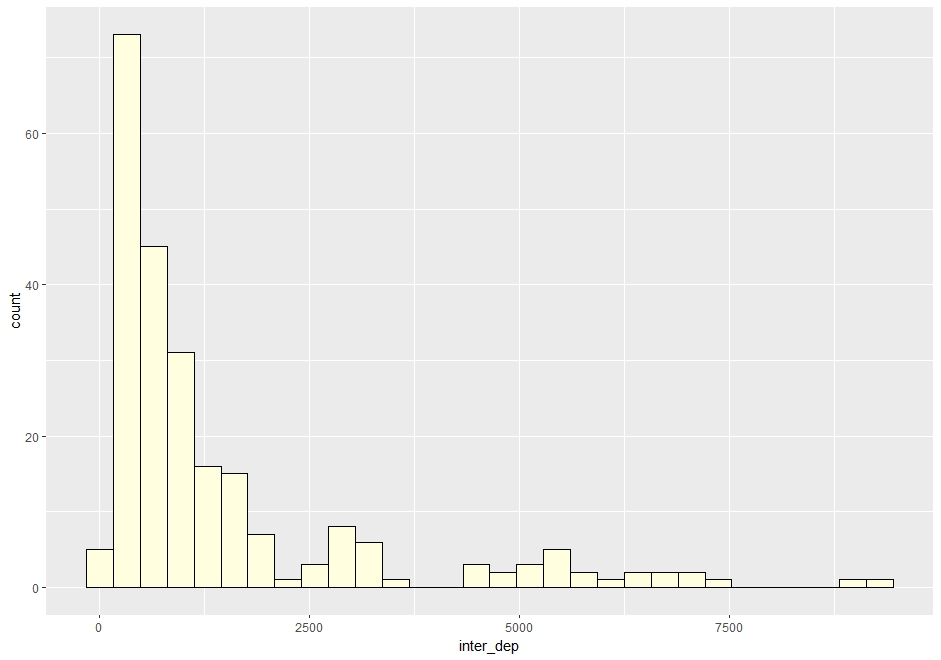I see inter_dep are alos right skewed.
That's it. Thank you!

Next post is

To read from the first post,